• English
• Account
• API
• Integrations
• Enterprise
• Resources
Comparing numeric values using IF in Airtable
• 07 Dec 2023
• Dark
Light
• PDF

# Comparing numeric values using IF in Airtable

• Dark
Light
• PDF

Article Summary

This article covers how to compare numeric values using the IF function in Airtable to streamline your team's and organization's work.

## Introduction

 Plan availability All plan types Platform(s) Web/Browser, Mac app, and Windows app Related reading Getting started with formulas

The IF function returns one of two values depending on whether a logical argument is true or not. This article will cover how to compare numeric values (numbers) against a logical argument using an IF statement.

IF statements (or conditional statements) are written in the following format:

``````IF([logical argument], [value1],  [value2])
``````

This function means "If [logical argument is true], then return [value1]. Otherwise, return [value2]." The logical argument can be either text or a value, e.g. `{Pet's Name}='Rufus' or {Test Score}>75.`

## Comparing values in Airtable

As an example, a purchasing manager for a bicycle shop needs to track the revenue generated by each item in stock. She needs to keep track of her purchase costs, retail price, profit margin, and markup percentage.

Profit margin can be calculated with this formula: `{Retail Price} - {Vendor Cost}`

While markup can be calculated with: `({Retail Price} - {Vendor Cost}) / {Vendor Cost}`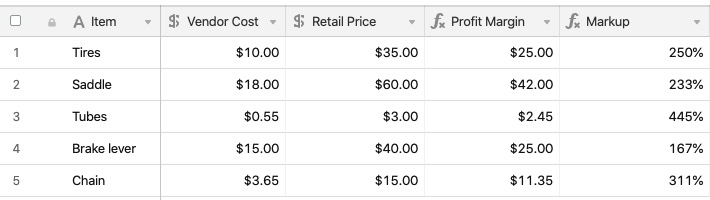To easily filter her table by how profitable each item can be, another formula can be added to identify the items with the highest profits margins (over 200%). Note in the formula below that when referencing a percentage field in a formula, you'll need to multiply the field values by 100 in order to maintain the properly formatted percent value.

``````IF(
({Markup}*100) >= 250,
"🏆 High profit margin",
"👍 Normal profit margin"
)
``````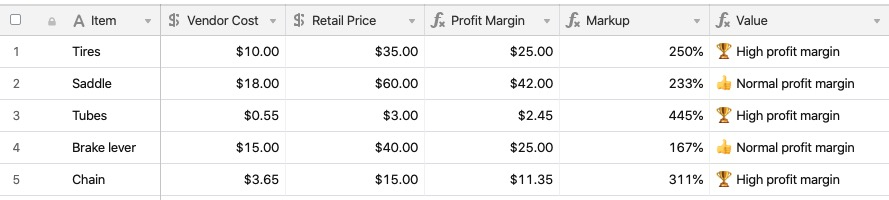The purchasing manager has some basic information just using these formulas, but to stay profitable she also wants to ensure that every item they sell is above a minimum markup percentage depending on the item.

Using the formula below, she can output a message based off of the Markup target listed for each item.

``````IF({Markup} > {Markup target},"✅ Profitable","❌ Increase price")
``````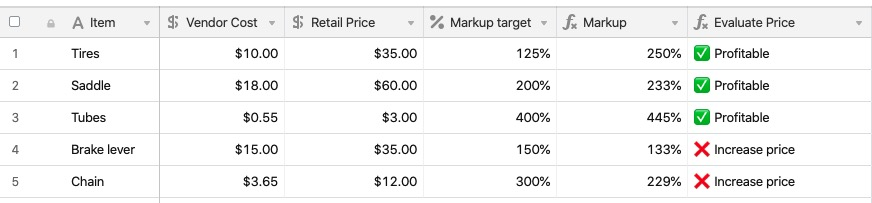## Advanced work with percentages in Airtable

While considering a storewide sale of 25% off, the purchasing manager needs to ensure that each item will remain profitable when discounted by the sale. She can use this formula in order to determine which items would be priced too low given the sale.

``````IF(
(Markup - {Sale Discount})*100>({Markup target}*100),
"✅ Okay for storewide sale",
"❌ Priced below profit margin"
)
``````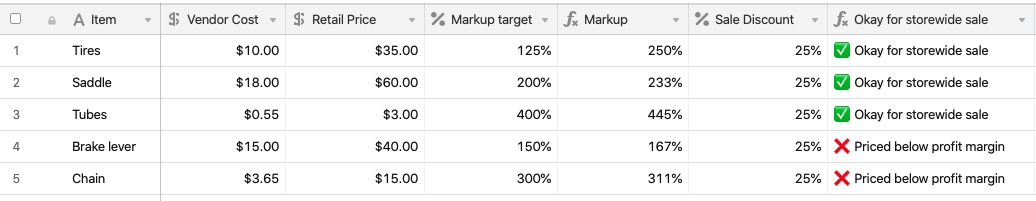The result of the formula is helpful, but could provide even more information to our purchasing manager to help inform the decision for the weekend sale. By adjusting the formula, you can add in logic to display how much the sale amount will need to be lowered in order for that item to remain profitable. Note that the formula below uses another new function - ROUND() - to round the displayed percentage to one decimal point.

``````IF(
(Markup - {Sale Discount})*100>({Markup target}*100),
"✅ Okay for storewide sale",
"❌ Decrease sale discount by at least "
&
ROUND(({Markup target}*100 - (({Markup}-{Sale Discount})*100)),1)
&
"%"
)
``````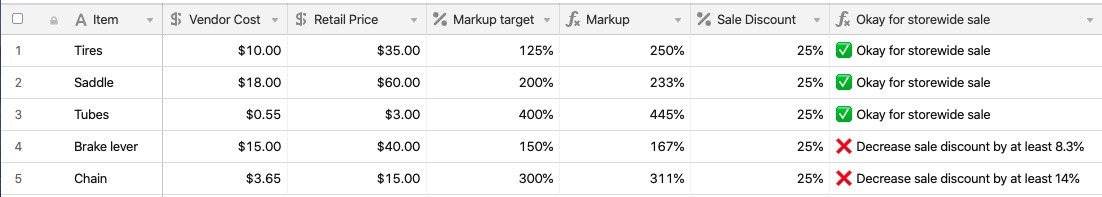With this information provided by the IF statement, the purchasing manager can adjust the sale discount to make sure both her customers - and her profits - are happy.